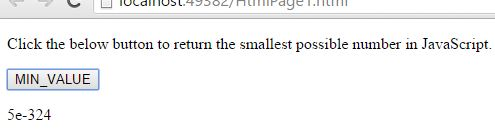# MIN_VALUE Property in JavaScript

##### How to return the smallest number possible in JavaScript?

By using `MIN_VALUE` property we can return the smallest number possible in JavaScript.

```<p>Click the below button to return the smallest possible number in JavaScript.</p>
<input type="button" value="MIN_VALUE" onclick="Function()" />
<p id="myId"></p>

<script>
function Function() {
document.getElementById("myId").innerHTML = Number.MIN_VALUE;
}
</script>
```

In the above code snippet we have given `Id` as "`myId`"to the second `<p>` element in the HTML code, we need to return the smallest number possible in JavaScript. For that we are using the code `Number.MIN_VALUE`. There is a Function() in the`<script>`block which is connected to the Onclick of the HTML button. The `document.getElementById(`"myId"`) ` returns the element that has Id "id=myID" in the HTML page. Onclick of the button "MIN_VALUE" fires the Function() in the `<script>` block at the same time `Number.MIN_VALUE `returns the smallest number possible in JavaScript and gives the output.

OUTPUTNOTE: In the above output the value of e=10, the `MIN_VALUE` is very closer to the zero(0). The number smaller than the `MIN_VALUE` are converted to 0 in JavaScript.

Views: 3269 | Post Order: 83